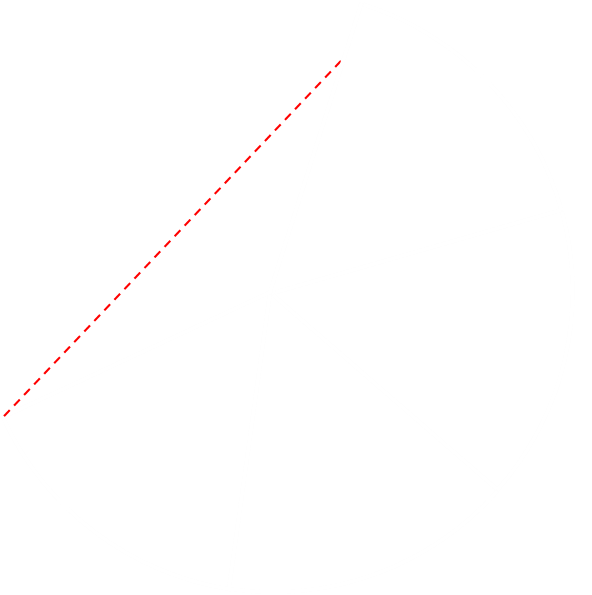# Christmas Tree Lights

Imagine you have a conical tree around which you would like to wrap a string of lights. Your goal is to wrap them around, in a helix pattern, starting at the bottom, and winding them up to the top.First let’s define our tree. The tree is a perfect cone, with a base radius of r, and a slant height of a. The circumference of the base of the tree is 2πr.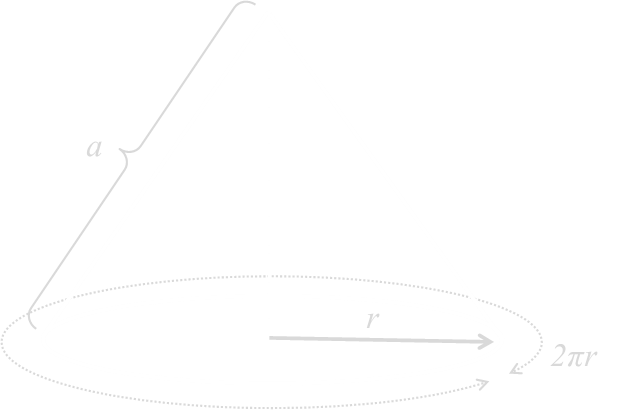If we 'cut' the cone up the slant diagonal, we can roll it flat to make a 2D mesh which is the sector of the a circle with a curved edge the same length as the circumference of the cone base. Depending on the dimensions and aspect ratio of the cone (ratio of the height to radius), the angle α can be derived. The relationship between these values is:## One Loop Around

Starting at the bottom of the tree, we'll take the string of lights, loop them around the tree once, raising them up so that after one complete loop we are at a position b above where we started. We'll then pull the string tight (this is important), removing all the slack. The question is, what is the shape of the curve made by the lights?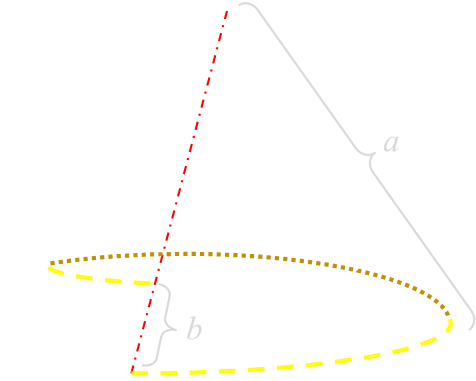The straightest distance between two points (in 2D Euclidean geometry) is a straight line. As we're pulling the string of lights tight (removing all slack), the locus of the lights is shown by the yellow line below in the flattened map of the cone.Using the Cosine rule, the length of the light string can be easily determined:So far this is all pretty vanilla, but have you noticed the very interesting thing yet?
As the taught line wraps around the cone, it initially goes 'up' the cone, but after a while, it reaches a maximum, then winds back 'down' to the end point! I don't know about you, but when I first saw this, it was counterintuitive.The lights wind up, then finish winding down!
We already known the length l, and we can define h to be the height of the peak of the locus from the top of the cone (this will also be a radius of the red circle, and perpendicular to the line), and u for the 'uphill' part of the wind, and d for the 'downhill' part of the wind.
There are two right triangles:We can subtract the top equation from the bottom, and rearrange to calculate the uphill length, and then substitute this back in to calculate the other values.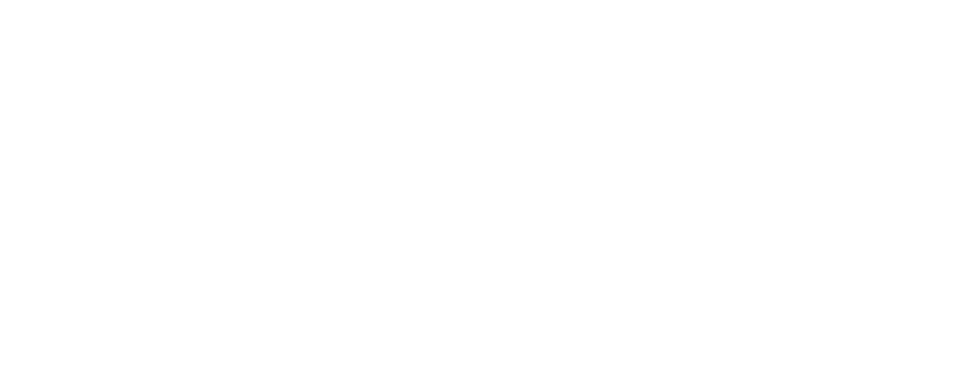• u is the length of the 'uphill' part of the string.
• d is the length of the 'downhill' part of the string.
• l is the total length of the string.
• h is the closest the string gets to the top of the tree.

## What does this look like?

Here's what is looks like if the loop difference is 20% of the slant slope:You can imagine pulling the string tight, and it forming this shape.

## More than one loop?

The above calculation was for the light string wrapping once around the tree. What if we wrap the lights around twice, and pulled them taught? How about three times?
Using the same geodesic principle, we can draw a straight line over the flattened sectors. This time, putting two or three sectors adjacent, for each loop. Pulling tight the string would wind up and down.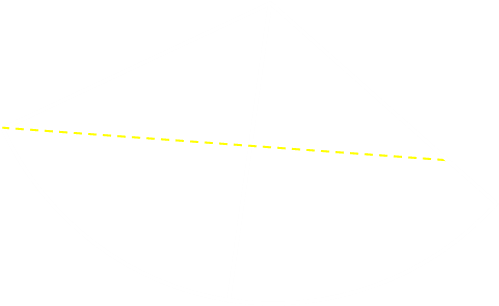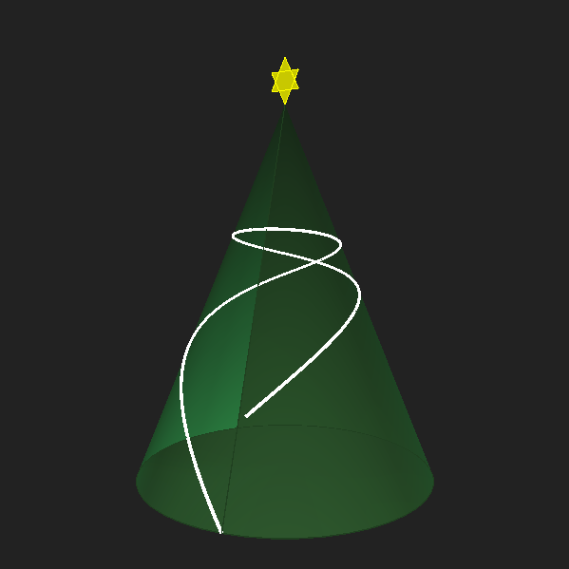However, when we get to four turns, we get to an impossible path. In this geometry, it's not possible to create a taught string.